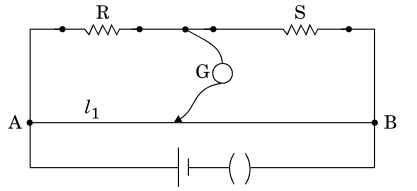NEET Physics Current Electricity Questions Solved

(a) Write the principle of working of a metro bridge.

(b) In a metre bridge, the balance point is found at a distance ${l}_{1}$ with resistances R and S as shown in the figure.An unknown resistance X is now connected in parallel to the resistance S and the balance point is found at a distance ${l}_{2}$. Obtain a formula for X in terms of .                                      (3)

Explanation is a part of a Paid Course. To view Explanation Please buy the course.

Difficulty Level: